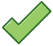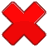## SQL SERVER | Find Number of Days in a Year

There are two types of Years – Leap year and Non Leap year. Lets first understand what are they-

Leap Year

• A normal year has 365 days.
• A Leap Year has 366 days (the extra day is the 29th of February).

## How to know if a year will be a Leap Year:Leap Years are any year that can be evenly divided by 4 (such as 2012, 2016, etc)except if it can can be evenly divided by 100, then it isn’t (such as 2100, 2200, etc)except if it can be evenly divided by 400, then it is (such as 2000, 2400)

Method 1 | Using Simple Maths & IIF/CASE Statements

 ``` /* Find no of days in a Year - Leap Year / Non Leap Year Every year has 365 days If the year is leap then we have to add 1 , so logic will be 365 + add 1 if the year is leap */ DECLARE @YEAR AS INT = 2012 SELECT NofDays = 365 + ISNULL(CASE WHEN @YEAR%400=0 THEN 1 WHEN @YEAR%4=0 THEN 1 WHEN @YEAR%100=0 THEN 0 END,0) GO DECLARE @YEAR AS INT = 2012 SELECT NofDays = 365 + IIF( @YEAR%400 =0 , 1 , IIF(@YEAR%4 =0, 1, IIF(@YEAR%100 = 0,0,0))) GO -- ```

Method 2 | Using Recursive CTE

 ``` -- /* Method 2 | Using Recursive CTE Note | We have to add OPTION (MAXRECURSION 366) If the above option is not sepecified below error will come "Msg 530, Level 16, State 1, Line 11 The statement terminated. The maximum recursion 100 has been exhausted before statement completion." */ DECLARE @YEAR AS INT = 2015 ;WITH CTE AS ( SELECT CAST(CONCAT('01/01/',@YEAR) AS DATETIME) y1 UNION ALL SELECT DATEADD(d,1,y1) y1 FROM CTE WHERE DATEADD(d,1,y1) <= CAST(CONCAT('12/31/',@YEAR) AS DATETIME) ) SELECT COUNT(*) NoOfDays FROM CTE OPTION (MAXRECURSION 366) -- ```

Method 3 | Using DateDiff

 ``` -- /* Method 3 | Using DateDiff and Cast/Convert */ DECLARE @YEAR AS INT = 2012 SELECT DATEDIFF(d,CAST(CONCAT('01/01/',@YEAR) AS DATETIME) ,CAST(CONCAT('12/31/',@YEAR) AS DATETIME) + 1) -- ```

Method 4 | Using New DATEFROMPARTS & DATEPART

 ``` -- /* Method 4 | Using New DATEFROMPARTS & DATEPART */ DECLARE @YEAR AS INT = 2012 SELECT NofDays = DATEPART(dy,DATEFROMPARTS(@YEAR,12,31)) -- ```